# R S Aggarwal Solutions for Class 11 Maths Chapter 19- Graphs of Trigonometric Functions

R S Aggarwal Solutions are useful for students as it helps them in scoring high marks in the examination. The R S Aggarwal Solutions given in this page contains detailed step-by-step explanations to all the problems that come under Chapter 19, Graphs of Trigonometric Functions, of the Class 11 R S Aggarwal Textbook. This chapter deals with the concept of Graphs of Trigonometric Functions.

These solutions are prepared by subject matter experts at BYJU’S, describing the complete method of solving problems. By understanding the concepts used in R S Aggarwal Solutions for Class 11 Maths, students will be able to clear all their doubts related to “Graphs of Trigonometric Functions”.

### Download PDF of R S Aggarwal Solutions for Class 11 Maths Chapter 19- Graphs of Trigonometric Functions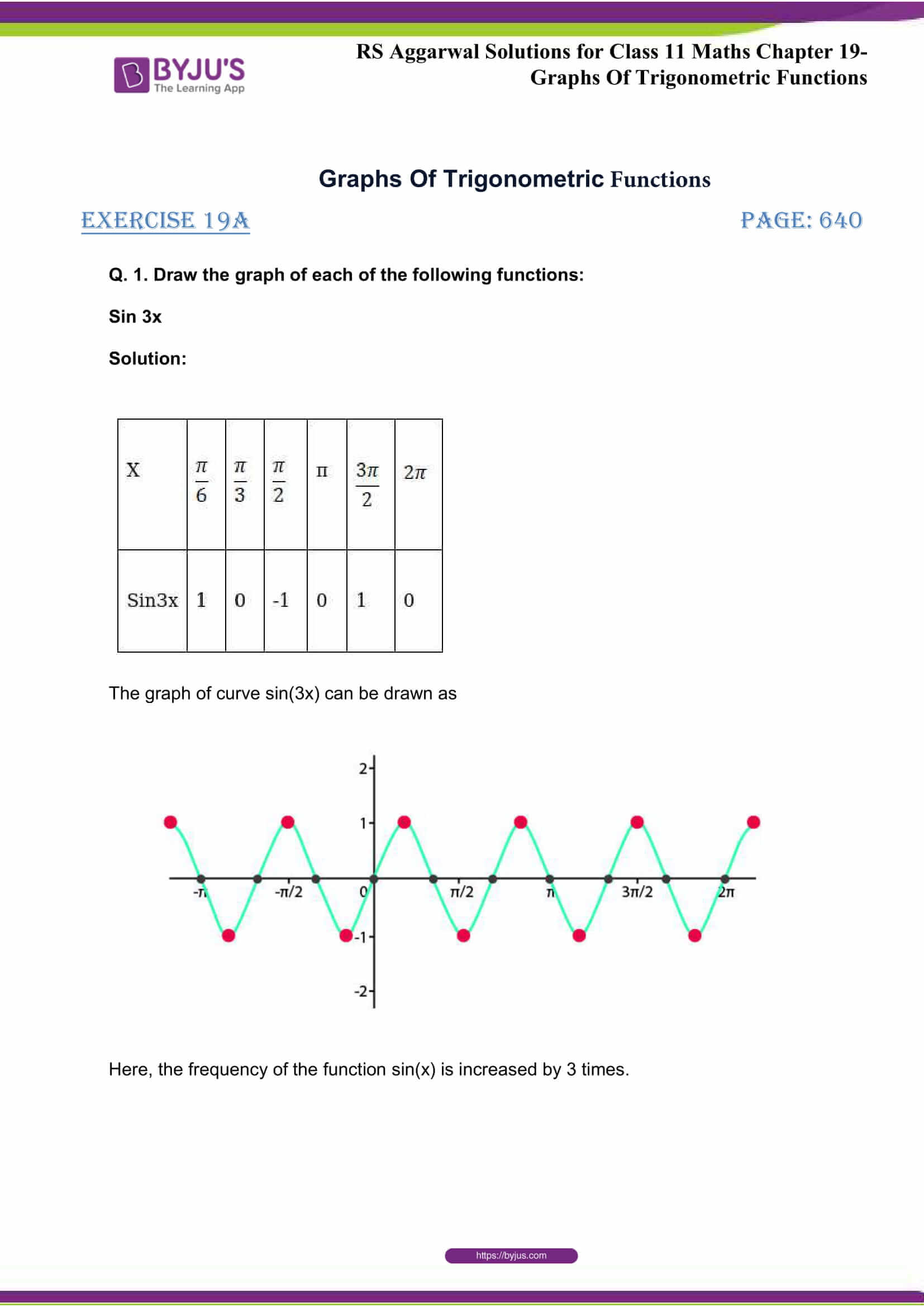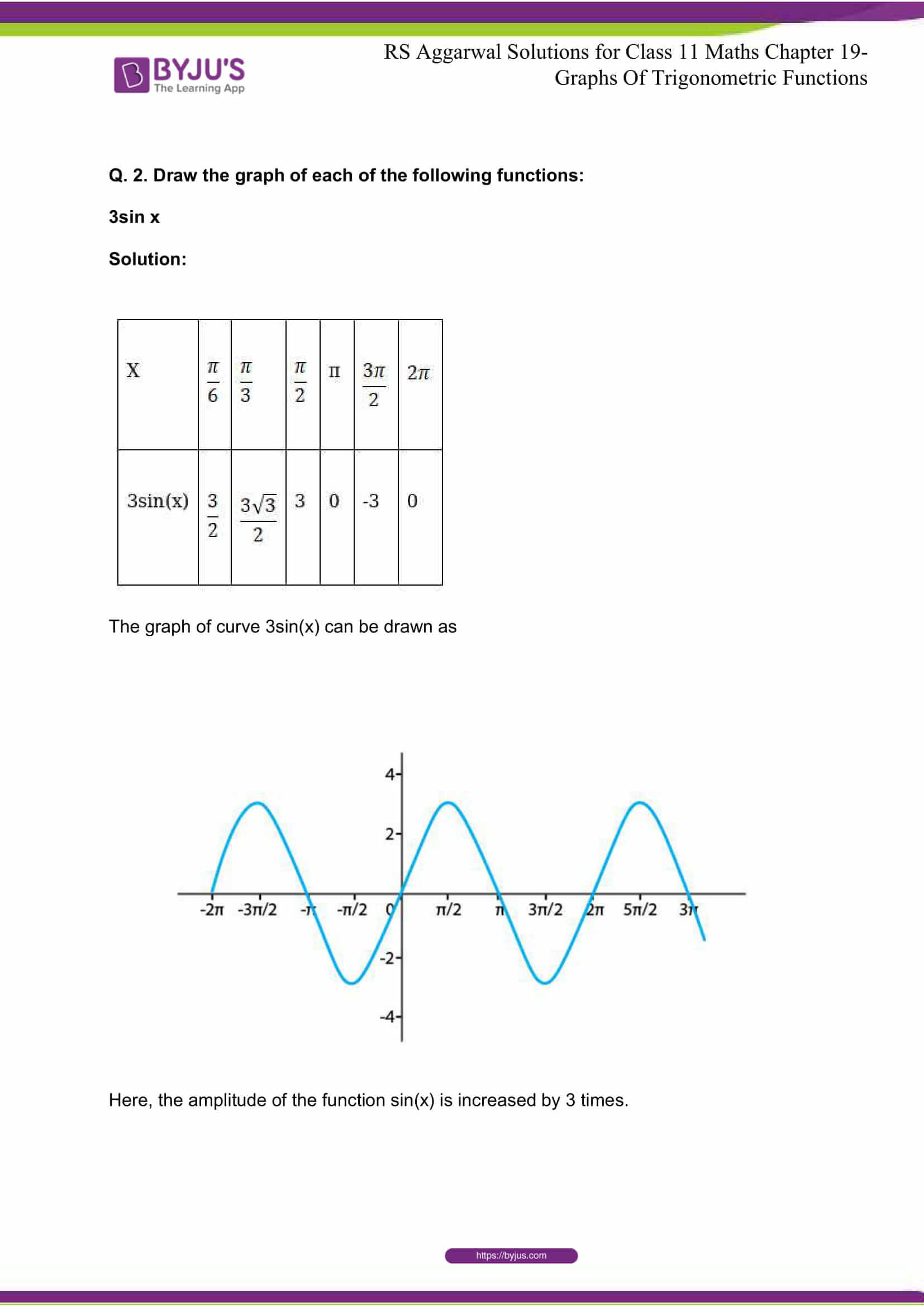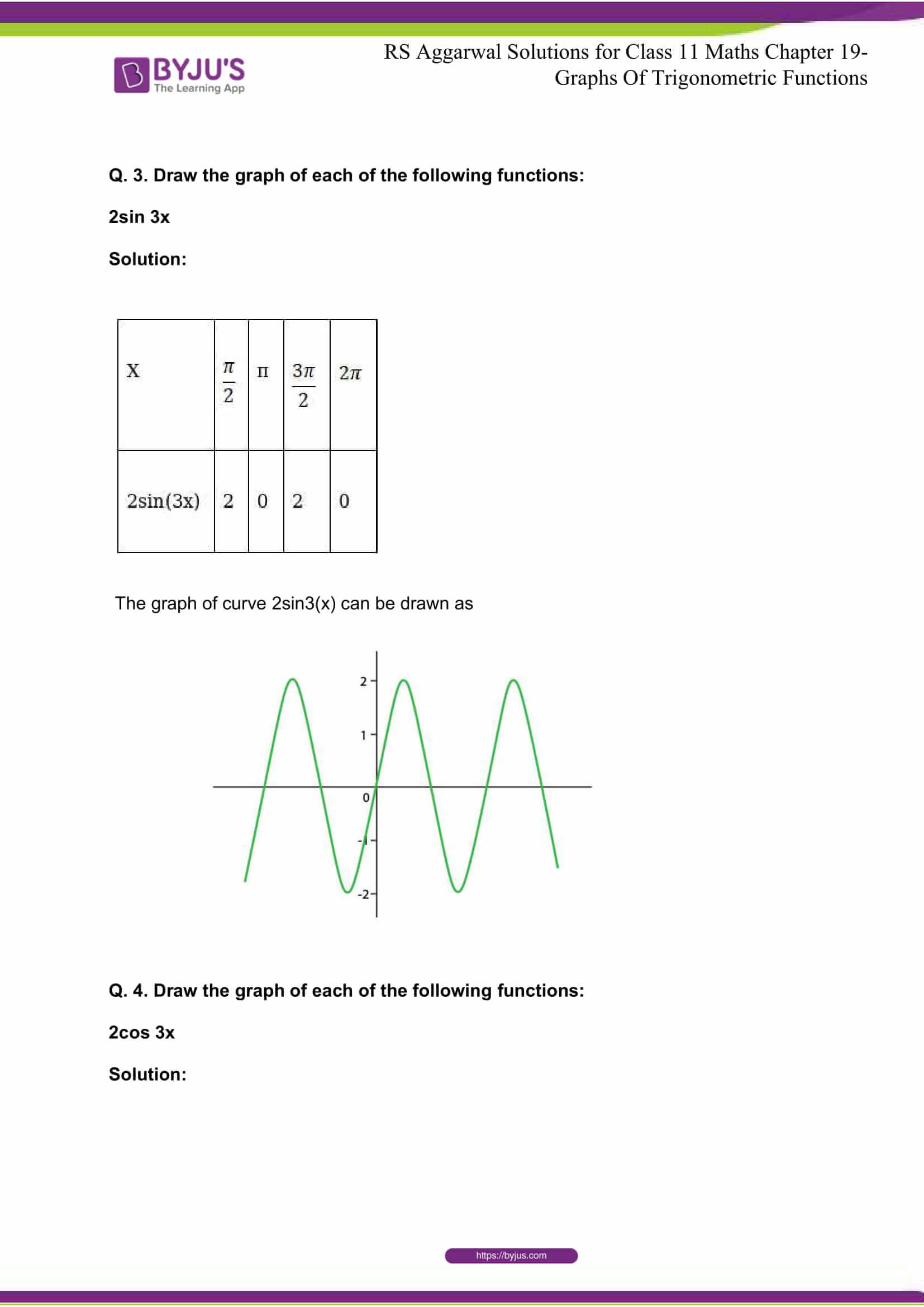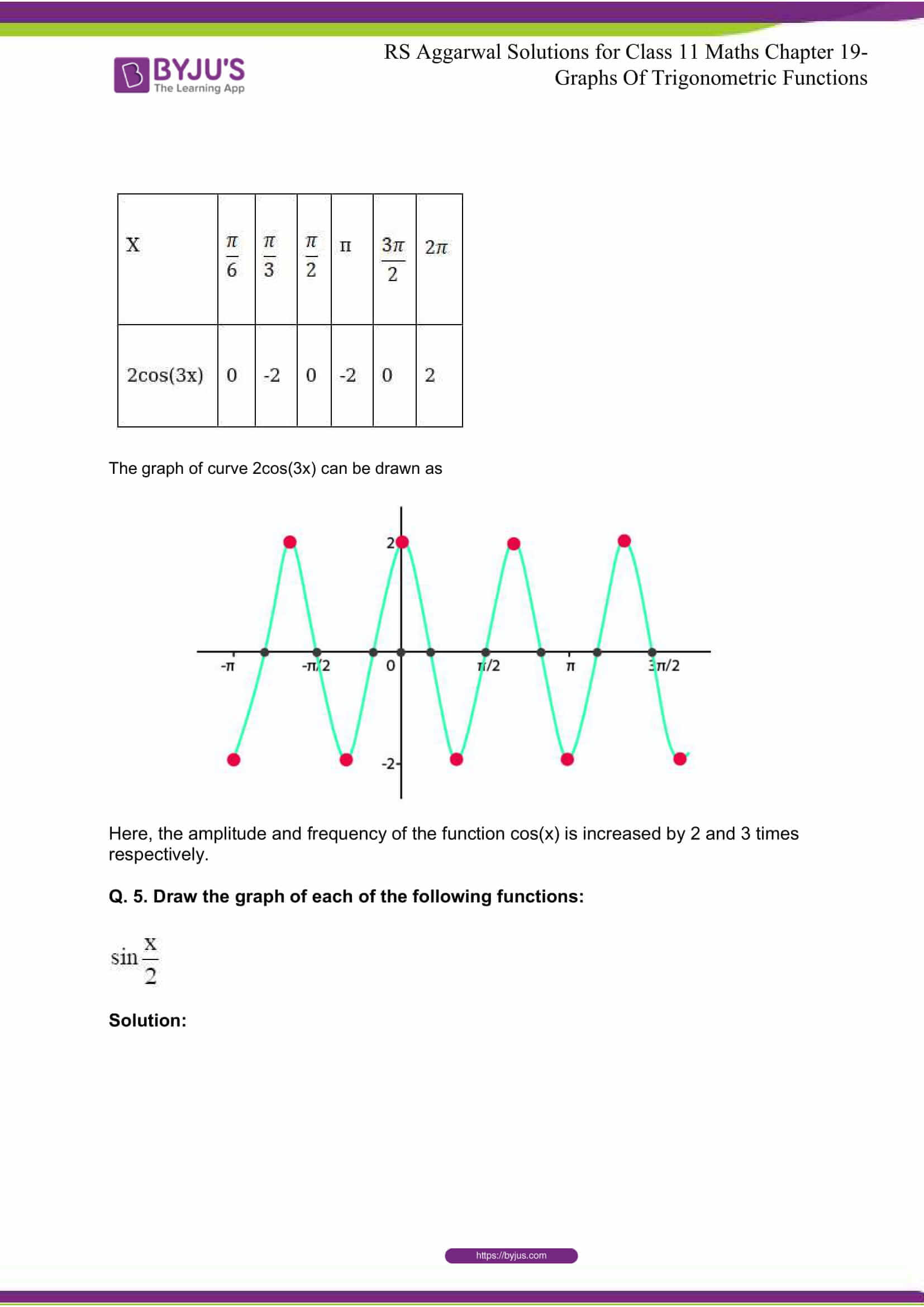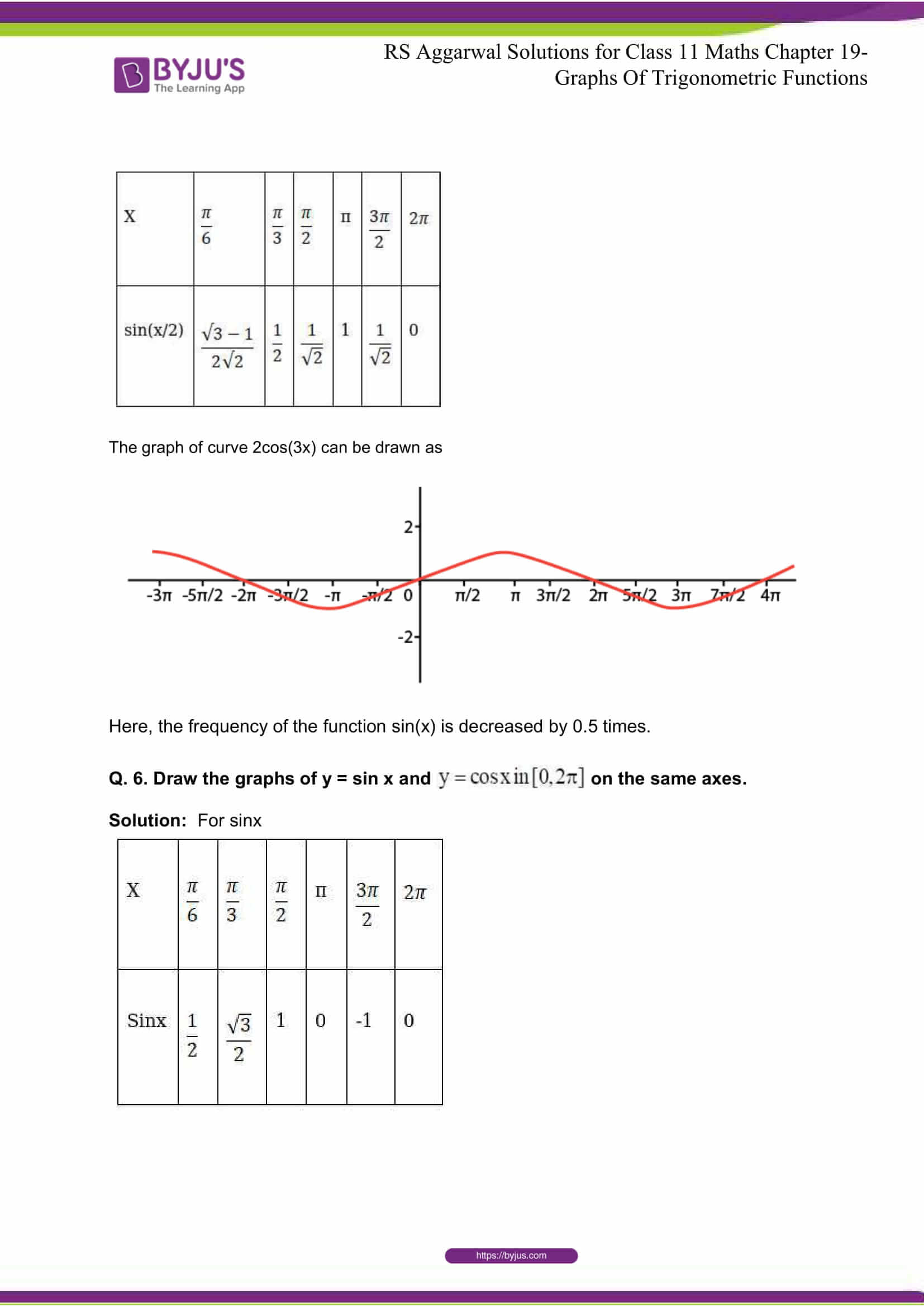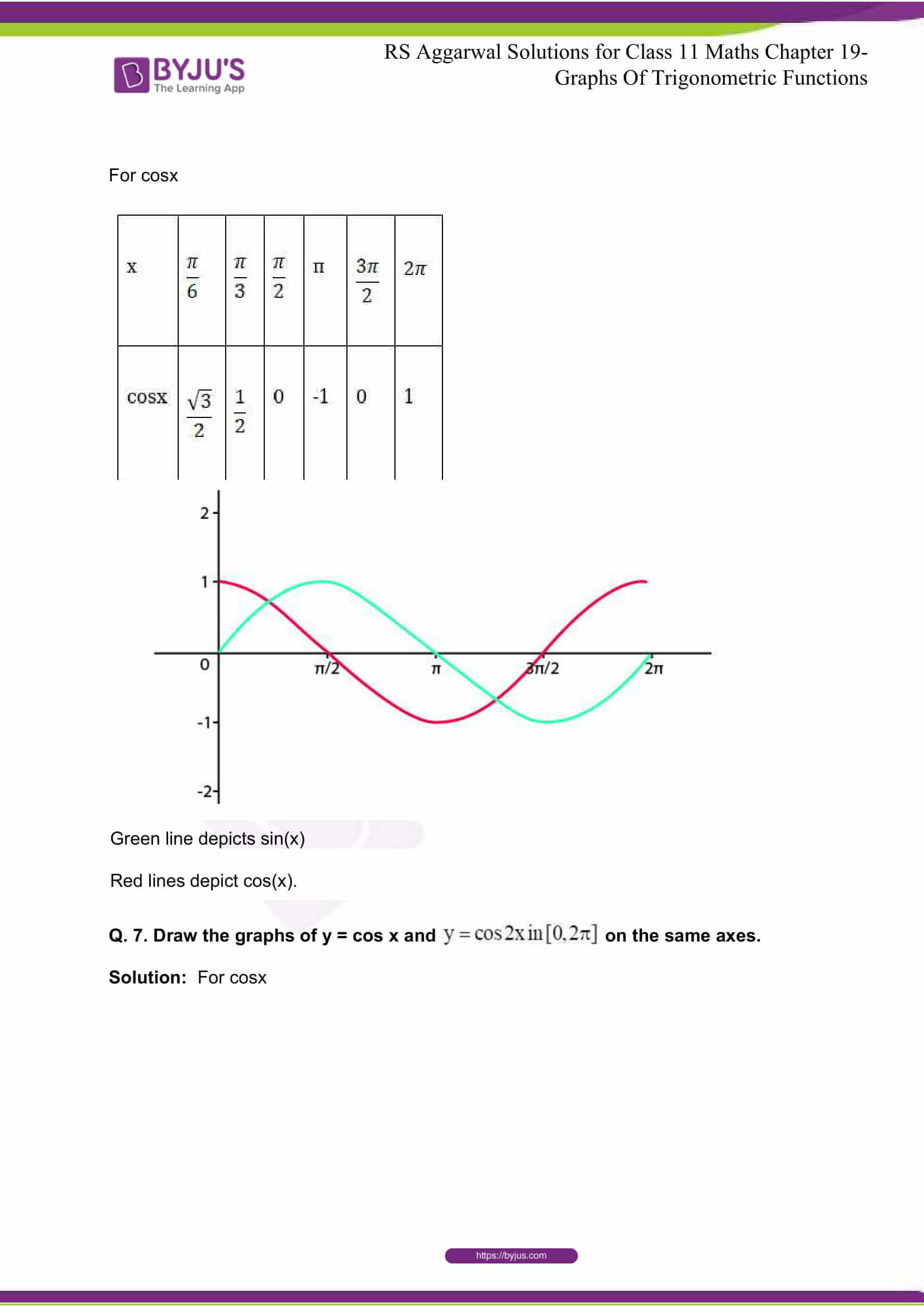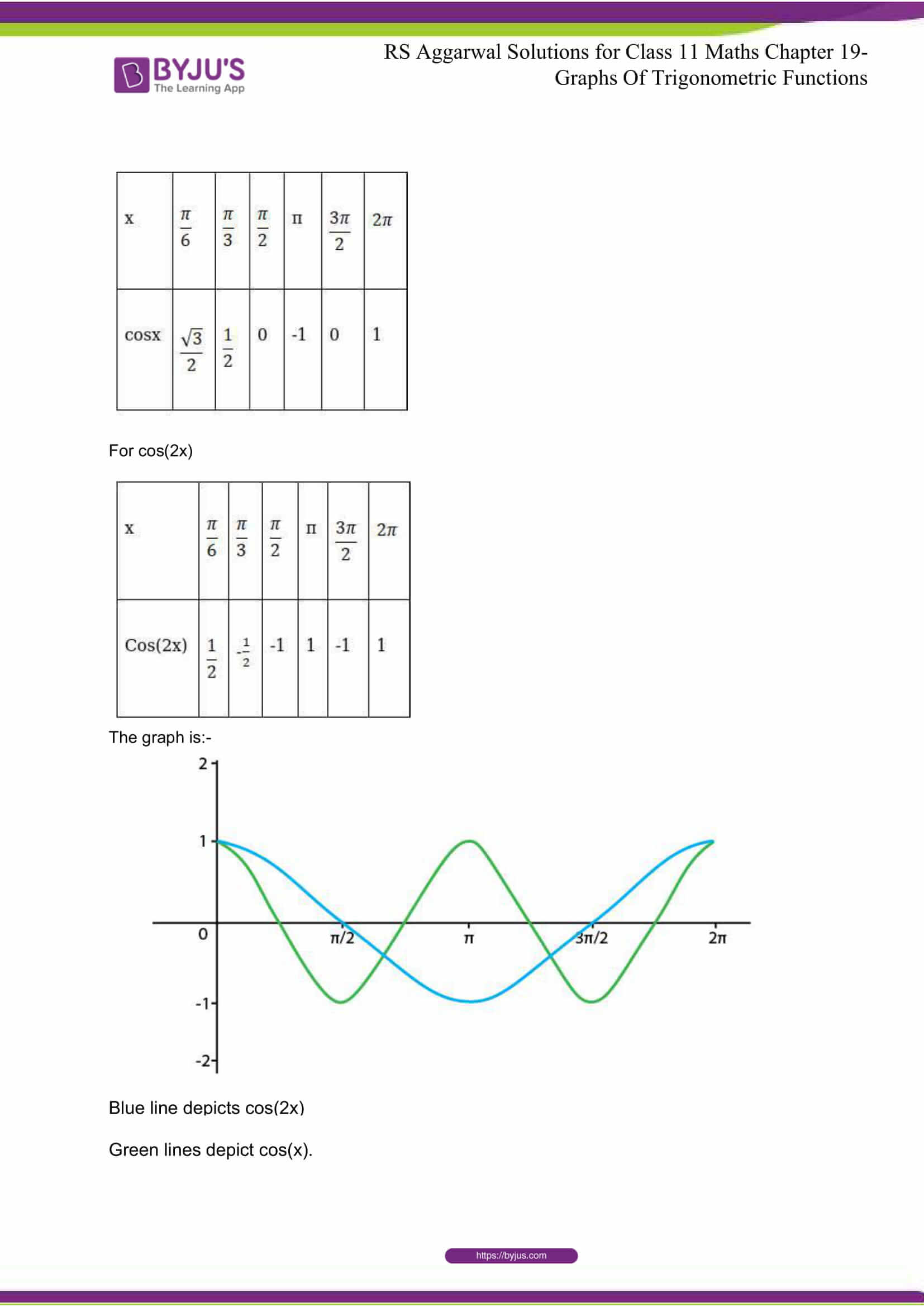## R S Aggarwal Solutions for Class 11 Maths Chapter 19- Graphs of Trigonometric Functions

Chapter 19, Graphs of Trigonometric Functions, is composed of 1 exercise and the solutions given here contain answers to all the questions present in this exercise. Let us have a look at some of the topics that are being discussed in this chapter.

19.1 Graph of y= sin x

19.2 Graph of y= cos x

19.3 Graph of y= a sin bx

19.4 Graph of y= a cos bx

## R S Aggarwal Solutions for Class 11 Maths Chapter 19- Graphs of Trigonometric Functions

Chapter 19 discusses and uses the concept related to the Graphs of Trigonometric Functions along with their applications. Read and learn the 19th chapter of R S Aggarwal textbook to get up to date with the concepts related to Graphs of Trigonometric Functions that are taught in the class. Learn the R S Aggarwal Solutions for Class 11 effectively to score high in the examination.